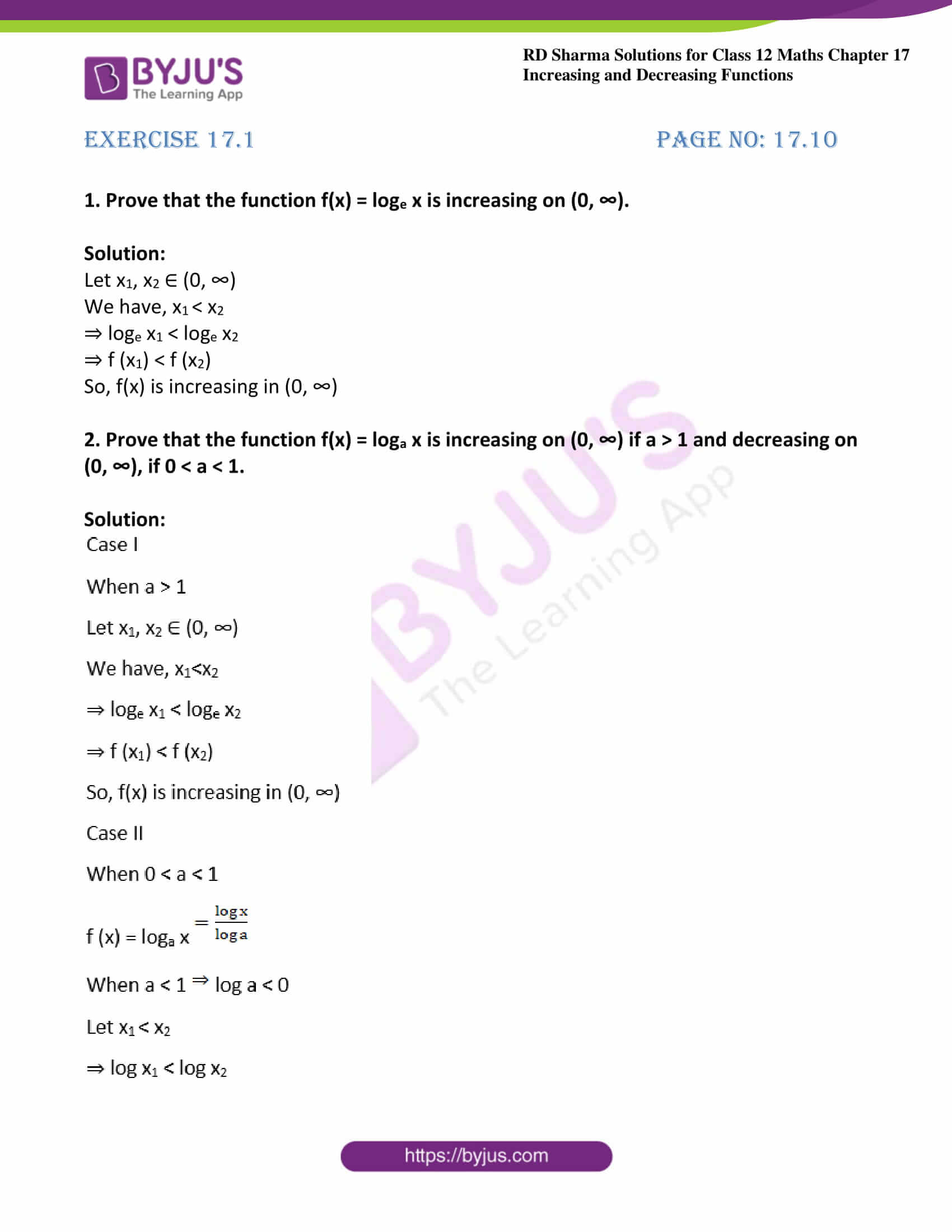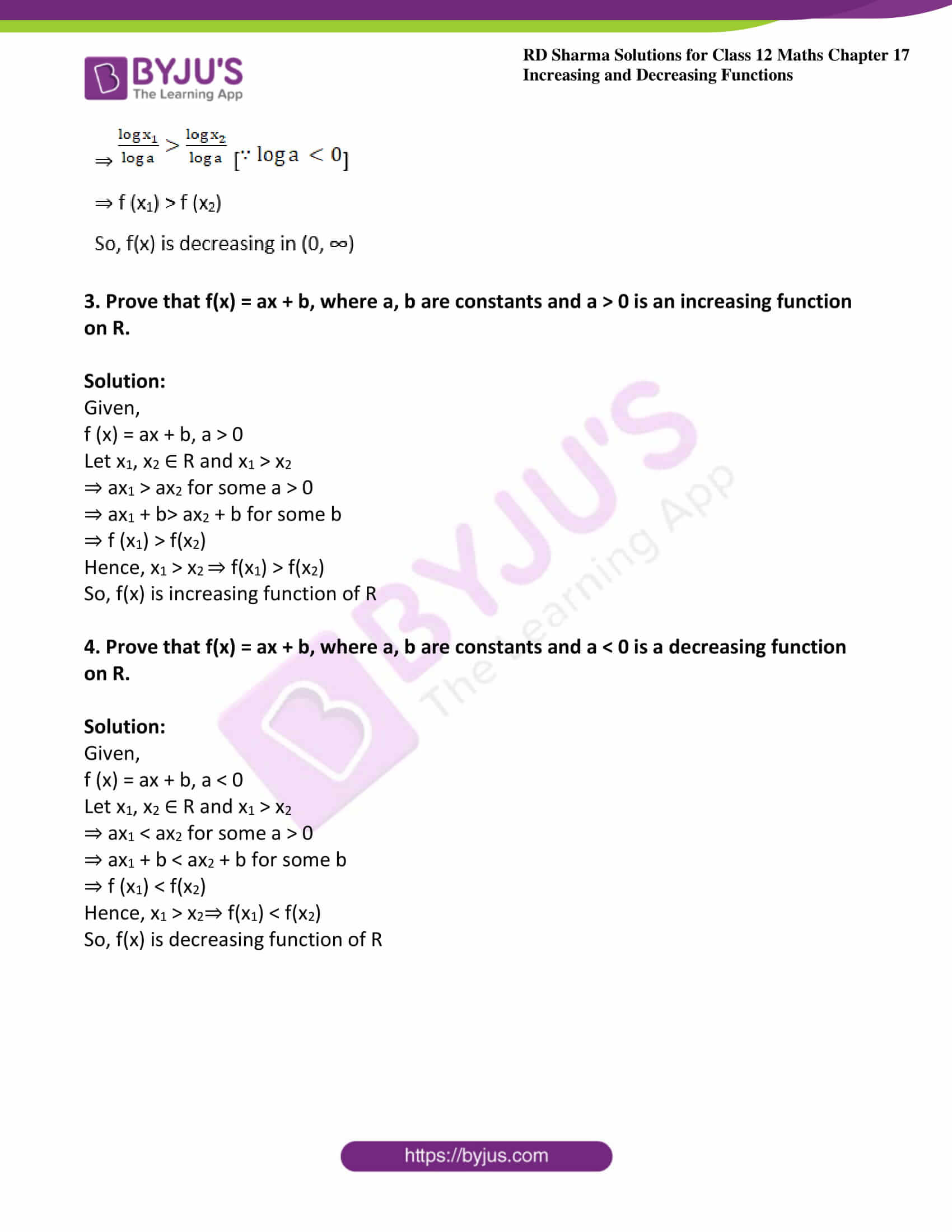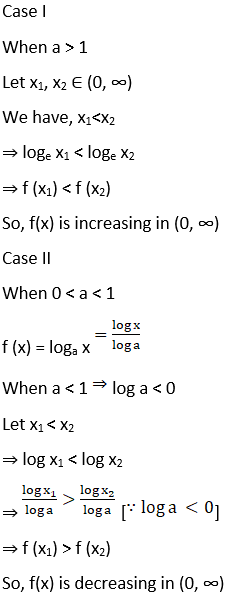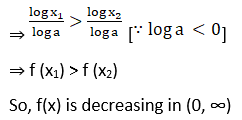# RD Sharma Solutions For Class 12 Maths Exercise 17.1 Chapter 17 Increasing and Decreasing Functions

RD Sharma Solutions for Class 12 Maths Exercise 17.1 Chapter 17 Increasing and Decreasing Functions are provided here. Strictly increasing, strictly decreasing and verification of increasing or decreasing functions are covered under this exercise. By practising RD Sharma Solutions for Class 12 Maths, students can improve their performance and work on their strengths and weaknesses.

The PDF of Chapter 17 Exercise 17.1 of RD Sharma Solutions are provided here. These solutions help them to a great extent. A set of experts at BYJU’S formulated these solutions to guide the students. Some of the important topics of this exercise are listed below.

• The solution of rational algebraic inequations
• Strictly increasing functions
• Strictly decreasing functions
• Monotonic functions
• Monotonically increasing function
• Monotonically decreasing functions

## RD Sharma Solutions Class 12 Maths Chapter 17 Increasing and Decreasing Functions Exercise 17.1:### Access another exercise of RD Sharma Solutions For Class 12 Chapter 17 – Increasing and Decreasing Functions

Exercise 17.2 Solutions

### Access answers to Maths RD Sharma Solutions For Class 12 Chapter 17 – Increasing and Decreasing Functions Exercise 17.1

Exercise 17.1 Page No: 17.10

1. Prove that the function f(x) = loge x is increasing on (0, ∞).

Solution:

Let x1, x2 ∈ (0, ∞)

We have, x1 < x2

⇒ loge x1 < loge x2

⇒ f (x1) < f (x2)

So, f(x) is increasing in (0, ∞)

2. Prove that the function f(x) = loga x is increasing on (0, ∞) if a > 1 and decreasing on (0, ∞), if 0 < a < 1.

Solution:3. Prove that f(x) = ax + b, where a, b are constants and a > 0 is an increasing function on R.

Solution:

Given,

f (x) = ax + b, a > 0

Let x1, x2 ∈ R and x1 > x2

⇒ ax1 > ax2 for some a > 0

⇒ ax1 + b> ax2 + b for some b

⇒ f (x1) > f(x2)

Hence, x1 > x2 ⇒ f(x1) > f(x2)

So, f(x) is increasing function of R

4. Prove that f(x) = ax + b, where a, b are constants and a < 0 is a decreasing function on R.

Solution:

Given,

f (x) = ax + b, a < 0

Let x1, x2 ∈ R and x1 > x2

⇒ ax1 < ax2 for some a > 0

⇒ ax1 + b < ax2 + b for some b

⇒ f (x1) < f(x2)

Hence, x1 > x2⇒ f(x1) < f(x2)

So, f(x) is decreasing function of R